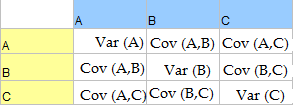# Matrices and Matrix Algebra

Share on

## Matrix Algebra: an Introduction

A matrix is a rectangular array of numbers arranged into columns and rows (much like a spreadsheet). Matrix algebra is used in statistics to express collections of data. For example, the following is an Excel worksheet with a list of grades for exams: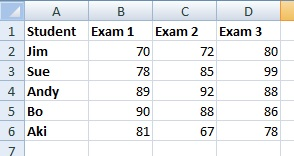Conversion to matrix algebra basically just involves taking away the column and row identifiers. A function identifier is added (in this case, “G” for grades):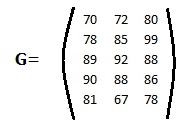Numbers that appear in the matrix are called the matrix elements.

## Matrices: Notation

Why the Strange Notation?
We use the different notation (as opposed to keeping the data in a spreadsheet format) for a simple reason: convention. Keeping to conventions makes it easier to follow the rules of matrix math (like addition and subtraction). For example, in elementary algebra, if you have a list like this: 2 apples, 3 bananas, 5 grapes, then you would change it to 2a+3b+5g to keep to convention.

Some of the most common terms you’ll come across when dealing with matrices are:

• Dimension (also called order): how many rows and columns a matrix has. Rows are listed first, followed by columns. For example, a 2 x 3 matrix means 2 rows and 3 columns.
• Elements: the numbers that appear inside the matrix.
• Identity matrix (I): A diagonal matrix with zeros as elements except for the diagonal, which has ones.
• A scalar: any real number.

## Matrix Algebra: Addition and Subtraction

The size of a matrix (i.e. 2 x 2) is also called the matrix dimension or matrix order. If you want to add (or subtract) two matrices, their dimensions must be exactly the same. In other words, you can add a 2 x 2 matrix to another 2 x 2 matrix but not a 2 x 3 matrix. Adding matrices is very similar just regular addition: you just add the same numbers in the same location (for example, add all numbers in column 1, row 1 and all numbers in column 2, row 2).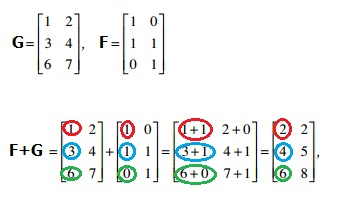A note on notation: A worksheet (for example, in Excel) uses column letters (ABCD) and row numbers (123) to give a cell location like A1 or D2. It’s typical for matrices to use notation like gij which means the ith row and jth column of matrix G.

Matrix subtraction works exactly the same way.

Matrix addition is just a series of additions. For a 2×2 matrix:

• Add the top left numbers together and write the sum in a new matrix, in the top left position.
• Add the top right numbers together and write the sum in the top right.
• Add the bottom left numbers together and write the sum in the bottom left.
• Add the bottom right numbers together and write the sum in the bottom right: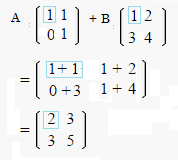Use exactly the same procedure for a 2×3 matrix: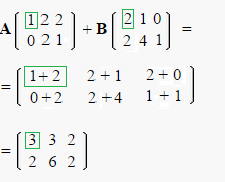In fact, you can use this basic technique for any matrix addition as long as your matrices have the same dimensions (the same number of columns and rows). In other words, if the matrices are the same size, you can add them. If they aren’t the same size, you can’t add them.

• A matrix with 4 rows and 2 columns can be added to a matrix with 4 rows and 2 columns.
• A matrix with 4 rows and 2 columns cannot be added to a matrix with 5 rows and 2 columns.

The above technique is sometimes called the “entrywise sum” as you’re simply adding entries together and noting the result.

### Another way to think about it…

Think about what a matrix represents. This very simple matrix [5 2 5] could represent 5x + 2y + 5z. And this matrix [2 1 6] could equal 2x + y + 6z. If you add them together using algebra, you would get:
5x + 2y + 5z + 2x + y + 6z = 7x + 3y + 11z.
This is the same result as you would get from adding the entries in the matrices together.

## Matrix Addition for Unequal Dimensions

If you have unequal dimensions, you can still add the matrices together, but you’d have to use a different (much more advanced) technique. One such technique is the direct sum. The direct sum (⊕)of any pair of matrices A of size m × n and B of size p × q is a matrix of size (m + p) × (n + q):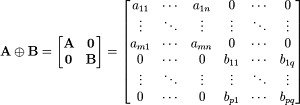For example:## Matrix Multiplication

It’s relatively easy to multiply by a single number (called a “scalar multiplication”), like 2: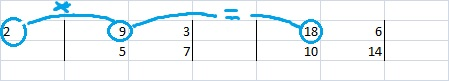Just multiply each number in the matrix by 2 and you get a new matrix. In the image above:
2 * 9 = 18
2 * 3 = 6
2 * 5 = 10
2 * 7 = 14

The results from the four multiplications produce the numbers in the new matrix on the right.

## Matrix Multiplication: Two Matrices

When you want to multiply two matrices together, the process becomes a little more complicated. You need to multiply the rows of the first matrix by the columns of the second matrix. In other words, multiply across rows of the first matrix and down columns of the second matrix. Once you’ve multiplied through, add the products and write out the answers as a new matrix.

If this all sounds a little complicated, this (very short) video shows how it’s done:

You can only perform matrix multiplication on two matrices if the number of columns in the first matrix equals the number of rows in the second matrix. For example, you can multiply a 2 x 3 matrix (two rows and three columns) by a 3 x 4 matrix (three rows and four columns).

Obviously this can become a very complex (and tedious) process. However, you can find many decent matrix multiplication tools online. I like this one by Matrix Reshish. After calculation, you can multiply the result by another matrix, and another, meaning that you can multiply many matrices together.

Microsoft Excel can also perform matrix multiplication using the “array” functions. You can find instructions here on the Stanford website. Scroll down to where it says Matrix Operations in Excel.

## Definition of a Singular Matrix

A quick look at a matrix can possibly tell you if it is a singular matrix. If the matrix is square and has one row or column of zeros or two equal columns or two equal rows, then it’s a singular matrix. For example, the following ten matrices are all singular (image: Wolfram):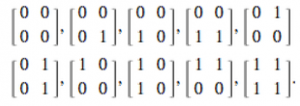There are other types of singular matrices, some are not quite so easy to spot. Therefore, a more formal definition is necessary.

The following three properties define a singular matrix:

1. The matrix is square and
2. It does not have an inverse.
3. It has a determinant of 0.

### 1. The Square Matrix

A square matrix has (as the name suggests) an equal number of rows and columns. In more formal terms, you would say a matrix of m columns and n rows is square if m=n. Matrices that are not square are rectangular.
A singular matrix is a square matrix, but not all square matrices are singular.

### Noninvertible Matrices

If a square matrix does not have an inverse, then it’s a singular matrix.

The inverse of a matrix is the same idea as a reciprocal of a number. If you multiple a matrix by its inverse, you get the identity matri, matrix equivalent of 1. The identity matrix is basically a series of ones and zeros. The identity matrix differs according the the size of the matrix.

If you’re unfamiliar with finding inverses, you may want to watch this short video:

### Determinant of Zero

A determinant is just a special number that is used to describe matrices and finding solutions to systems of linear equations. The formula for calculating a determinant differs according to the size of the matrix. For example, a 2×2 matrix, the formula is ad-bc.

This simple 2×2 matrix is singular because its determinant is zero: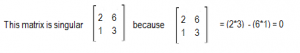## What is an Identity Matrix?

An identity matrix is a square matrix with 1s as the elements in the main diagonal from top left to bottom right and zeros in the other spaces. When you multiply a square matrix by an identity matrix, it leaves the original square matrix unchanged. For example: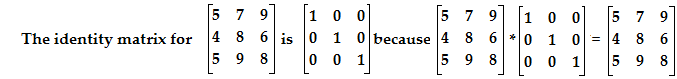The idea is similar to the identity element. In basic math, the identity element leaves a number unchanged. For example, in addition the identity element is 0, because 1 + 0 = 1, 2 + 0 = 2 etc. and in multiplication, the identity element is 1 because any number multiplied by 1 equals that number (i.e. 10 * 1 = 10). In more formal terms, if x is a real number, then the number 1 is called the multiplicative identity because 1 * x = x and x * 1 = x. By the same logic, the identity matrix I gets it’s name because, for all matrices A, I * A = A and A * I = A.

In matrix algebra, the identity element is different depending on the size of the matrix you are operating on; unlike the singular 1 for the multiplicative identity and 0 for additive identity, there is no single identity matrix for all matrices. For any n * n matrix there is an identity matrix In * n. The main diagonal will always have 1s and the remaining spaces will all be zeros. The following image shows identity matrices for a 2 x 2 matrix and a 5 x 5 matrix: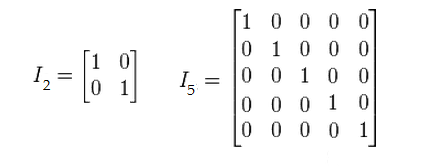When people talk about the “Identity Matrix” they are usually talking about the multiplicative identity matrix. However, there is another type: the additive identity matrix. When this matrix is added to another, you end up the original matrix. Not surprisingly, every element in these matrices are zeros. Therefore, they are sometimes called the zero matrix.

## What is an Inverse Matrix?

Inverse matrices are the same idea as reciprocals. In elementary algebra (and perhaps even before that), you came across the idea of a reciprocal: one number multiplied by another can equal 1.

If you multiply one matrix by it’s inverse, you get the matrix equivalent of 1: the Identity Matrix, which is basically a matrix with ones and zeros.

Finding an inverse matrix involves quite a few steps. Watch this short video on how to find an inverse matrix or follow the steps below:

Step 1: Find the adjugate of the matrix. The adjugate of the matrix can be found by rearranging one diagonal and taking negatives of the other: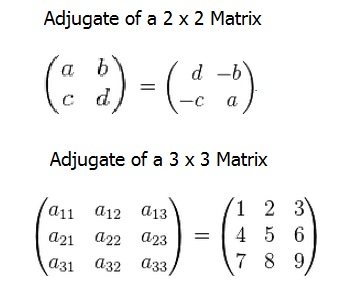To find the adjugate of a 2×2 matrix, swap diagonals a and d, then swap the signs of c and d.

Step 2: Find the determinantof the matrix. For a matrix
A B C D (see above image), the determinant is (a*d)-(b*c).
Step 3: Multiply 1/determinant * adjugate. .

You can check your answer with matrix multiplication. Multiply your answer matrix by the original matrix and you should get the identity matrix. You can also use the online calculator here.

## What is an Eigenvalue?

An eigenvalue (λ) is a special scalar used in matrix multiplication and is of particular importance in several areas of physics, including stability analysis and small oscillations of vibrating systems. When you multiply a matrix by a vector and get the same vector as an answer, along with a new scalar, the scalar is called an eigenvalue. The basic equation is:
Ax = λx; we say that λ is an eigenvalue of A.
All the above equation is saying is that if you take a matrix A and multiply it by vector x, you get the exact same thing as if you take an eigenvalue and multiply it by vector x.

## Eigenvalue Example

In the following example, 5 is an eigenvalue of A and (1,2) is an eigenvector: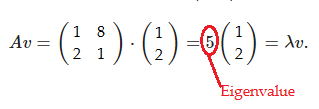Let’s take a look at this in steps, to demonstrate visually exactly what an eigenvalue is. In general multiplication, if you multiply an n x n matrix by an n x 1 vector, you get a new n x 1 vector as a result. This next image shows this principle for a 2 x 2 matrix multiplied by (1,2):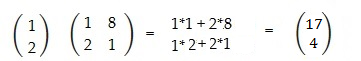What if, instead of a new n x 1 matrix, it was possible to get an answer with the same vector you multiplied by, along with a new scalar?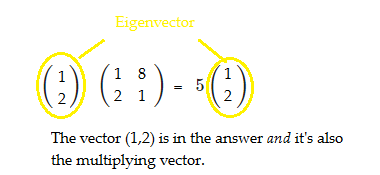When this is possible, the multiplying vector (i.e. the one that’s in the answer as well) is called an eigenvector and the corresponding scalar is the eigenvalue. Note that I said “when this is possible”, because sometimes it isn’t possible to calculate a value for λ. The decomposition of a square matrix A into eigenvalues and eigenvectors (it’s possible to have multiple values of these for the same matrix) is known in called eigen decomposition. Eigen decomposition is always possible if the matrix consisting of the eigenvectors of A is square.

## Calculating

Find the eigenvalues for the following matrix: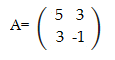Step 1: Multiply the identity matrix by λ. The identity matrix for any 2×2 matrix is [1 0; 0 1], so: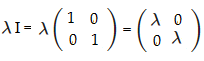Step 2: Subtract your answer from Step 1 from the matrix A using matrix subtraction: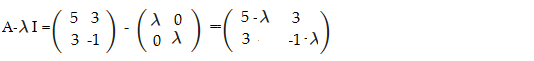Step 3: Find the determinant of the matrix you calculated in Step 2:
det = (5-λ)(-1-λ) – (3)(3)
Simplifying, we get:
-5 – 5λ + λ + λ2 – 9
= λ2 – 4λ – 14

Step 4: Set the equation you found in Step 3 equal to zero and solve for λ:
0 = λ2 – 4λ – 14 = 2
I like to use my TI-83 to find the roots, but you could also use algebra or this online calculator. Finding the roots (zeros), we get x = 2 + 3√2, 2 – 3√2

Answer: 2 + 3√2 and 2 – 3√2

The math for larger matrices is the same, but the calculations can get very complex. For 3×3 matrices, use the calculator at the bottom of this section; for larger matrices, try this online calculator.

## What is an Augmented Matrix?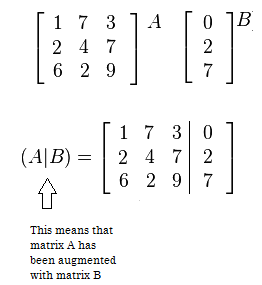The image above shows an augmented matrix (A|B) on the bottom. Augmented matrices are usually used to solve systems of linear equations and in fact, that’s why they were first developed. The three columns on the left of the bar represent the coefficients (one column for each variable). This area is called the coefficient matrix. The last column to the right of the bar represents a set of constants (i.e. values to the right of the equals sign in a set of equations). It’s called an augmented matrix because the coefficient matrix has been “augmented” with the values after the equals sign.

For example, the following system of linear equations:

x + 2y + 3z = 0
3x + 4y + 7z = 2
6x + 5y + 9z = 11

Can be placed into the following augmented matrix: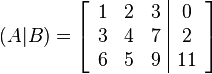One you have put your system into an augmented matrix, you can then perform row operations to solve the system.

You don’t have to use the vertical bar in an augmented matrix. It’s common to see matrices without any lines at all. The bar just makes it easier to keep track of what your coefficients are and what your constants to the right of the equals sign are. Whether you use a vertical bar at all depends on the textbook you’re using and your instructor’s preference.

## Writing a System of Equations

You can also work backwards to write a system of linear equations given an augmented matrix.
Sample question: Write a system of linear equations for the following matrix.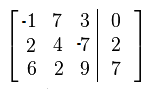Step 1:Write the coefficients for the first column followed by “x”. Make sure to note positive or negative numbers:
-1x
2x
6x
Step 2:Write the coefficients for the second column, followed by “y.” Add if it’s a positive number, subtract if it’s negative:
-1x + 7y
2x + 4y
6x + 2y
Step 3:Write the coefficients for the second column, followed by “z.” Add if it’s a positive number, subtract if it’s negative:
-1x + 7y + 3
2x + 4y – 7
6x + 2y + 9
Step 3:Write the constants in the third column, preceded by an equals sign.
-1x + 7y + 3 = 0
2x + 4y – 7 = 2
6x + 2y + 9 = 7
Note: if you have a negative sign in this step, just make the constant a negative number.

## What is the Determinant of a Matrix?

The determinant of a matrix is just a special number that is used to describe matrices for finding solutions to systems of linear equations, finding inverse matrices and for various applications in calculus. A definition in plain English is impossible to pin down; it’s usually defined in mathematical terms or in terms of what it can help you do. The determinant of a matrix has several properties:

• It is a real number. This includes negative numbers.
• Determinants only exist for square matrices.
• An inverse matrix only exists for matrices with non-zero determinants.

The symbol for the determinant of a matrix A is |A|, which is also the same symbol used for absolute value, although the two have nothing to do with each other.

The formula for calculating the determinant of a matrix differs according to the size of the matrix.

## Determinant of a 2×2 matrix

The formula for the determinant of a 2×2 matrix is ad-bc. In other words, multiply the upper left element by the lower right, then subtract the product of the upper right and lower left.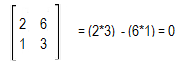## Determinant of a 3×3 matrix

The determinant of a 3×3 matrix is found with the following formula:
|A| = a(ei – fh) – b(di – fg) + c(dh – eg)
This may look complicated, but once you’ve labeled the elements with a,b,c on the top row, d,e,f on the second row and g,h,i on the last, it becomes basic arithmetic.
Example:
Find the determinant of the following 3×3 matrix: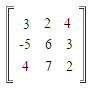=3(6×2-7×3)–5(2×2-7×4)+4(2×3-6×4)
=-219
What’s basically going on here is that you’re multiplying a,b, and d by the determinants of smaller 2x2s within the 3×3 matrix. This pattern continues for finding determinants of higher order matrices.

## Determinant of a 4×4 matrix

In order to find the determinant of a 4×4 matrix, you’ll first need to find the determinants of four 3×3 matrices that are within the 4×4 matrix. As a formula: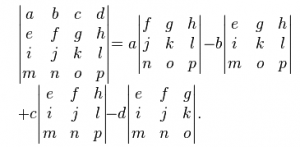## What is a Diagonal Matrix?

A diagonal matrix is a symmetric matrix with all zeros except for the leading diagonal, which runs from the top left to the bottom right.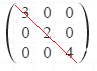The entries in the diagonal itself can also be zeros; any square matrix with all zeros can still be called a diagonal matrix.

The identity matrix, which has all 1s in the diagonal, is also a diagonal matrix. Any matrix with equal entries in the diagonal (i.e. 2,2,2 or 9,9,9), is a scalar multiple of the identity matrix and can also be classified as diagonal.

A diagonal matrix has a maximum of n numbers that are not zero, where n is the order of the matrix. For example, a 3 x 3 matrix (order 3) has a diagonal consisting of 3 numbers and a 5 x 5 matrix (order 5) has a diagonal of 5 numbers.

## Notation

The notation commonly used to describe the diagonal matrix is diag(a,b,c), where abc represents the numbers in the leading diagonal. For the above matrix, this notation would be diag(3,2,4)..

## Upper and Lower Triangular Matrices

The diagonal of a matrix always refers to the leading diagonal. The leading diagonal in a matrix helps to define two other types of matrix: lower-triangular matrices and upper triangular matrices. A lower-triangular matrix has numbers beneath the diagonal; an upper-triangular matrix has numbers above the diagonal.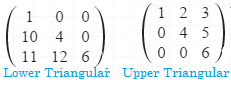A diagonal matrix is both a lower-diagonal and a lower-diagonal matrix.

## Rectangular Diagonal Matrices

For most common uses, a diagonal matrix is a square matrix with order (size) n. There are other forms not commonly used, like the rectangular diagonal matrix. This type of matrix also has one leading diagonal with numbers and the rest of the entries are zeros. The leading diagonal is taken from the largest square within the non-square matrix.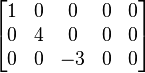## What is a Transpose Matrix?

A transpose matrix (or matrix transpose) is just where you switch all of the rows of the matrix into columns. Transpose matrices are useful in complex multiplication.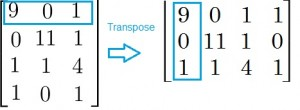An alternate way of describing a transpose matrix is that an element at row “r” and column “c” is transposed to row “c” and column “r.” For example, an element in row 2, column 3 would be transposed to column 2, row 3. The dimension of the matrix also changes. For example, if you had a 4 x 5 matrix you would transpose to a 5 x 4 matrix.

A symmetric matrix is a special case of a transpose matrix; it is equal to it’s transpose matrix.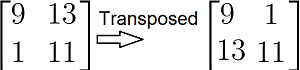In more formal terms, A = AT.

## Symbols for Transpose Matrix

The usual symbol for a transpose Matrix is AT However, Wolfram Mathworld states that two other symbols are use as well: A and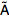.

## Properties of Transpose Matrices

Properties for transpose matrices are similar to the basic number properties that you encountered in basic algebra (like associative and commutative). The basic properties for matrices are:

• (AT)T = A: the transpose of a transpose matrix is the original matrix.
• (A + B)T = AT + BT: The transpose of two matrices added together is the same as the transpose of each individual matrix added together.
• (rA)T = rAT: when a matrix is multiplied by a scalar element, it doesn’t matter which order you transpose in (note:a scalar element is a quantity that can multiply a matrix).
• (AB)T = BT AT: the transpose of two matrices multiplied together is the same as the product of their transpose matrices in reverse order.
• (A-1)T = (AT)-1: the transpose and the inverse of a matrix can be performed in any order.

## What is a Symmetric Matrix?

A symmetric matrix is a square matrix that has symmetry around its leading diagonal, from top left to bottom right. Imagine a fold in the matrix along the diagonal (don’t include the numbers in the actual diagonal). The top right half of the matrix and the bottom left half are mirror images about the diagonal: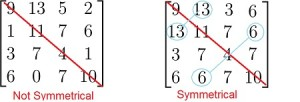If you can map the numbers to each other along the line of symmetry (always the leading diagonal), like the example on the right, you have a symmetrical matrix.

## Alternate Definition

Another way to define a symmetric matrix is that a symmetric matrix is equal to it’s transpose. A transpose of a matrix is where the first row becomes the first column, the second row becomes the second column, the third row becomes the third column…and so on. You’re basically just turning the rows into columns.If you take a symmetric matrix and transpose it, the matrix will look exactly the same, hence the alternate definition that a symmetric matrix is equal to its transpose. In mathematical terms, M=MT, where MT is the transpose matrix.

## Maximum Amount of Numbers

As most of the numbers in a symmetric matrix are duplicated, there is a limit to the amount of different numbers it can contain. The equation for the maximum amount of numbers in a matrix of order n is: n(n+1)/2. For example, in a symmetric matrix of order 4 like the one above there is a maximum of 4(4+1)/2 = 10 different numbers. This makes sense if you think about it: the diagonal is four numbers, and if you add up the numbers at the bottom left half (excluding the diagonal), you get 6.

## Diagonal Matrices

A diagonal matrix is a special case of a symmetric matrix. The diagonal matrix has all zeros except for the leading diagonal.

## What is a Skew Symmetric Matrix?

A skew symmetric matrix, sometimes called an antisymmetric matrix, is a square matrix that is symmetric about both diagonals. For example, the following matrix is skew symmteric: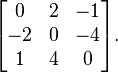Mathematically, a skew symmetric matrix meets the condition aij=-aji. For example, take the entry in row 3, column 2, which is 4. It’s symmetrical counterpart is the -4 in row 2, column 3. This condition can also be written in terms of its transpose matrix: AT=-A. In other words, a matrix is skew-symmetric only if AT=-A, where ATit the transpose matrix.

All of the leading diagonal entries in a skew symmetric matrix must be zero. This is because ai,i=−ai,i implies ai,i=0.

Another interesting property of the this type of matrix is that if you have two skew symmetrical matrices A and B of the same size, then you also get a skew symmetric matrix if you add them together:

This fact can assist you in proving that two matrices are skew-symmetric. The first step is to observe that all the entries in the leading diagonal are zero (something which would be impossible to “prove” mathematically!). The second step is adding the matrices together. If the result is a third matrix that is skew-symmetric, then you have proved that aij = – aji.

## Skew-Hermitian

A skew-Hermitian matrix is essentially the same as a skew symmetric matrix, except that the skew-Hermitian can contain complex numbers.

In fact, skew-symmetric and skew-Hermitian are equivalent for real matrices (a matrix that is composed almost entirely of real numbers).
The leading diagonal on a skew-Hermitian matrix must contain purely imaginary numbers; in the imaginary realm, zero is considered to be an imaginary number.

## What is a Variance-Covariance Matrix?

A variance-covariance matrix (also called a covariance matrix or dispersion matrix) is a square matrix that displays the variance and covariance of two sets of bivariate data together. The variance is a measure of how spread out the data is. Covariance is a measure of how much two random variables move together in the same direction.

The variances are displayed in the diagonal elements and the covariances between the pairs of variables are displayed in the off-diagonal elements. The variances are in the diagonals of the covariate matrix because basically, those variances are the covariates of each individual variable with itself.

The following matrix shows the variance for A (2.00), B (3.20) and C (0.21) in the diagonal elements.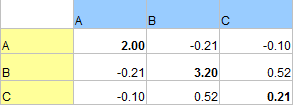The covariances for each pair are shown in the other cells. For example, the covariance for A and B is -0.21 and the covariance for A and C is -0.10. You can look in the column and row, or the row and column (i.e. AC or CA) to get the same result, because the covariance for A and C is the same as the covariance for C and A. Therefore, the variance-covariance matrix is also a symmetric matrix.

## Making a Variance-Covariance Matrix

Many statistical packages, including Microsoft Excel and SPSS, can make a variate-covariate matrix. Note that Excel calculates covariance for a population (a denominator of n) instead of for a sample (n-1). This can lead to slightly incorrect calculations for the variance-covariance matrix. To fix this, you’ll have to multiply each cell by n/n-1.

If you want to make one by hand:
Step 1: Insert the variances for your data into the diagonals of a matrix.
Step 2: Calculate the covariance for each pair and enter them into the relevant cell. For example, the covariance for A/B in the above example appears in two places (A B and B A). The following chart shows where each covariance and variance would appear for each option.